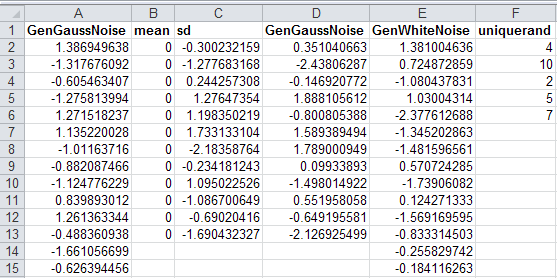# Random numbers generator in Excel

Although Excel’s Data Analysis Toolpak can generate good random numbers, I had to create these various functions to meet my specific needs.

## First: Generating Unique Random Numbers

This function was modified from ozgrid’s RandLotto function, which returns a string of numbers without duplicates. This function will return an array of unique random integers that are between the specified range. To return more than one number, enter this function as an array formula with Ctrl + Shift + Enter.

```Function UniqueRand(lngSmallest As Long, lngHighest As Long, HowManyNos As Long) As Long() 'modified from http://www.ozgrid.com/VBA/RandomNumbers.htm Dim iArr As Variant Dim i As Integer Dim r As Integer Dim temp As Integer Application.Volatile ReDim iArr(lngSmallest To lngHighest) For i = lngSmallest To lngHighest iArr(i) = i Next i For i = lngHighest To lngSmallest + 1 Step -1 r = Int(Rnd() * (i - lngSmallest + 1)) + lngSmallest temp = iArr(r) iArr(r) = iArr(i) iArr(i) = temp Next i Dim resultArr() As Long: ReDim resultArr(HowManyNos - 1, 0) For i = lngSmallest To lngSmallest + HowManyNos - 1 resultArr(j, 0) = iArr(i) j = j + 1 Next i UniqueRand = resultArr End Function```

## Second: Generating Gaussian or Normal Random Numbers with specified mean and Standard deviation(SD).

Now, this function is different from the Analysis toolpak utility, because the utility generates random numbers using a fixed mean and standard deviation. This function will, however, allow the user to generate random numbers with a different mean and SD.

```'This function will generate Gaussian or Normal Random Numbers based on given mean and SD Public Function GenGaussNoise(mean As Double, SD As Double, HowMany As Long) As Double() Dim x As Double, i As Long, j As Long itr = 50 Dim dblResArr() As Double: ReDim dblResArr(HowMany - 1, 0) For j = 1 To HowMany x = 0 For i = 1 To itr Randomize U = Rnd x = x + U Next i ' /* for uniform randoms in [0,1], mu = 0.5 and var = 1/12 */ ' /* adjust X so mu = 0 and var = 1 */ x = x - itr / 2 ' /* set mean to 0 */ x = x * Sqr(12 / itr) ' /* adjust variance to 1 */ dblResArr(j - 1, 0) = mean + SD * x Next j GenGaussNoise = dblResArr End Function```

## Third: Generate white noise or Guassian or Normal random numbers with mean = 0 and SD =1.

Although this could be achieved by the previous function, this function uses Box-Muller method. As with the previous function, you can use different mean and SD, but would default to mean =0 and SD=1.

```Public Function GenWhiteNoise(HowMany As Long, Optional mean As Double = 0, Optional variance As Double = 1) As Double() 'Returns a normally distributed random variate with mean 0 and variance 1, a.k.a. "White Noise". ' Uses the Box-Muller method Dim Value1 As Single, Value2 As Single, Fac As Single, Rsq As Single, SD As Double Dim dblResArr() As Double: ReDim dblResArr(HowMany - 1, 0) SD = Sqr(variance) For i = 1 To HowMany Do Randomize Value1 = 2 * Rnd - 1 Value2 = 2 * Rnd - 1 Rsq = Value1 ^ 2 + Value2 ^ 2 Loop Until Rsq > 0 And Rsq < 1 Fac = (-2 * Log(Rsq) / Rsq) ^ 0.5 If Rnd > 0.5 Then dblResArr(i - 1, 0) = mean + SD * Value1 * Fac Else dblResArr(i - 1, 0) = mean + SD * Value2 * Fac End If Next i GenWhiteNoise = dblResArr End Function```

## Example

You can see this random number generator in action.Random number generator

#### About the Author

The author of Tableau Data Visualization Cookbook and an award winning keynote speaker, Ashutosh R. Nandeshwar is one of the few analytics professionals in the higher education industry who has developed analytical solutions for all stages of the student life cycle (from recruitment to giving). He enjoys speaking about the power of data, as well as ranting about data professionals who chase after “interesting” things. He earned his PhD/MS from West Virginia University and his BEng from Nagpur University, all in industrial engineering. Currently, he is leading the data science, reporting, and prospect development efforts at the University of Southern California.

•Mark Laister says:

Hi Ashutosh,
Your white noise generator would be ideal for me to test some code I’m working on but when I try to use it, I get compiler errors on the lines “Loop Until Rsq > 0 And Rsq < 1” and “If Rnd < 0.5 Then”.
I’m afraid I am a VB illiterate. I am running Excel 2010. Can you offer any help?

Many thanks

Mark

•n.ashutosh says:

Hi, Mark,

Most likely, the code on this article got messed up. If you see >, you need to replace it with greater than sign. Similarly, < with a less than sign. I updated the article; try again. Save this function in your Excel file, and use it as a formula. You will need to use `Ctrl + Shift + Enter` to enter the formula.